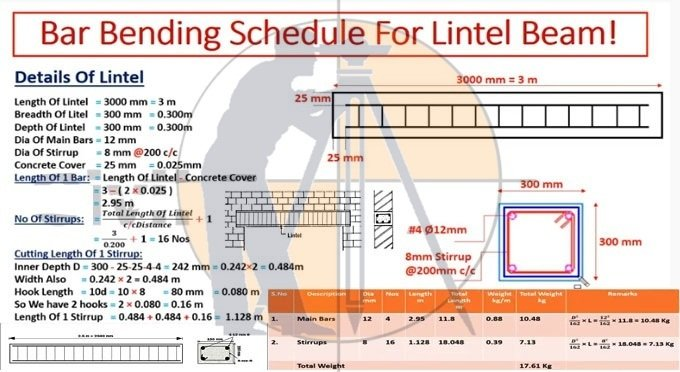Breaking News
Home / Civil Engineering / Bar Bending Schedule / Bar Bending Schedule Of Lintel Beam With Full Detail

# Bar Bending Schedule Of Lintel Beam With Full Detail

## Bar Bending Schedule Of Lintel Beam With Full Detail

Bar Bending Schedule is actually a chart made and utilized for calculating reinforcement and steel for slab, column and beam.Length of lintel = 3000 mm = 3 m

Breadth of lintel = 300 mm = 0.300 m

Lintel depth = 300 mm = 0.300 m

Concrete cover = 25 mm

Column width = 300 mm

Depth = 300 mm

Dia of main bars = 12 mm

There are 4 number main bars

Dia of steel of stirrup = 8 mm

### HOW TO CALCULATE STEEL QUANTITY FOR SLAB WITH BAR BENDING SCHEDULE

200 mm center to center space is between two stirrups

Dia of stirrup = 8 mm @ 200 c/c

One bar length = Lintel length – Concrete cover = 3 – (2 x 0.025) = 2.95 m

Number of stirrups = Overall length of lintel/center to center distance + 1

Number of stirrups = Overall length of lintel/c/c distance + 1

= 3/0.200 + 1 = 16

### One stirrup cutting length.

Internal/Inner depth, D = 300 – 25 – 25 – 4 – 4 = 242 mm = 0.242 (in m) x 2 = 0.484 m (as there are 2 sides)

Width = 0.242 x 2 = 0.484 m

Hook length = 10d = 10 x 8 = 80 mm = 0.080 m

As there are two hooks, 2 x 0.080 = 0.16 m

One stirrup length = 0.484 + 0.484 + 0.16 = 1.128 m

Description Diameter(mm) No. Length(m) Total length(m) Weight
(kg/m)
Total
weight(kg)
Remarks
Main bars 12 4 2.95 11.8 0.88 10.48 D2/162 x L = (12)2/162 x 11.8 = 10.48 kg
Stirrups 8 16 1.128 18.048 0.39 7.13 D2/162 x L = (8)2/162 x 18.048 = 7.13 kg
Total weight 17.61 kg

## . Land Surveying & Architects

### THANKS.